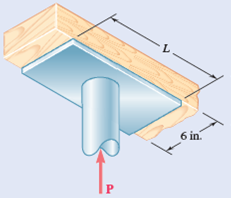Problem

# The axial force in the column supporting the timber beam shown is P = 20 kips. Determine t...

The axial force in the column supporting the timber beam shown is P = 20 kips. Determine the smallest allowable length L of the bearing plate if the bearing stress in the timber is not to exceed 400 psi.

Fig. P8.18#### Step-by-Step Solution

Solution 1

Since the bearing stress in the beam, should not exceed 400 psi,

$$\sigma_{\max } \leq 400$$ psi

Substitute $$\frac{P}{A}$$ for $$\sigma_{\max }$$.

$$\frac{P}{A} \leq 400 \mathrm{psi} \ldots \ldots$$ (1)

Here, $$P$$ is the applied axial force, and $$A$$ is the area of the bearing plate.

Calculate the area of the bearing plate.

$$A=L \times b$$

Here, $$L$$ is the smallest allowable length of the bearing plate and $$b$$ is the width of the plate.

Substitute 6 in. for $$b$$.

$$A=L \times 6$$

$$=6 L$$ in. $$^{2}$$

Calculate the smallest allowable length of the bearing plate.

Substitute $$6 L$$ in. $$^{2}$$ for $$A$$ and 20 kip for $$P$$ in equation (1).

$$\frac{P}{A} \leq 400 \mathrm{psi}$$

$$\frac{20 \times 10^{3} \mathrm{lb}}{6 L \text { in. }^{2}} \leq 400 \mathrm{psi}$$

$$\frac{20 \times 10^{3}}{6 L} \leq 400$$

$$L \geq \frac{20 \times 10^{3}}{6 \times 400}$$

$$L \geq 8.33$$ in.

For the beam to be under safe condition, the length of the beam should be greater than or equal to $$8.33 \mathrm{in}$$. but not less than $$8.33$$ in. Hence the smallest length of the beam is $$8.33$$ in.

$$L=8.33$$ in.

Therefore, the smallest allowable length of the timber beam is $$8.33 \mathrm{in}$$.